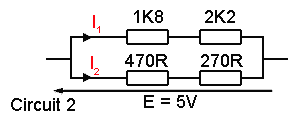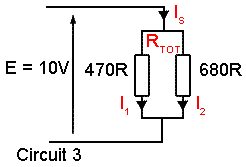# Network Calculations Quiz

## Voltages & Currents in Resistor Networks

Ohms law calculations and a few basic facts such the Potential Divider rule are really useful tools to work out voltages, currents and resistances in resistor networks, and are essential skills in understanding how circuits work and, when fault finding, understanding why they don´t work! Build your skills by working out some unknown values in the circuits below. Before you try this quiz however, it may be a good idea to look at some the basic facts about currents and voltages in resistor networks and some Ohms Law basics.

Use the tips given in our Series & Parallel Resistors and Ohms Law pages, work out your answers with pencil, paper and calculator.Then check your answers against the choices below.

### 1.

What is the value of the supply current Is in Circuit 1?### 2.

What is the value of the component current I1 in circuit 2?### 3.

Calculate the value of I2 in Circuit 2

### 4.

Calculate the total resistance RTOT of Circuit 3### 5.

What is the value of I1 in Circuit 3?

### 6.

Calculate the value of I2 in Circuit 3

### 7.

What is the supply current IS in Circuit 3?

### 8.

Calculate the potential difference VR2 across R2 in Circuit 4### 9.

In Circuit 4, what is the value of the supply current IS?

### 10.

In Circuit 4, what is the value of R2?